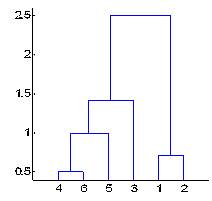## What is Clustering?

Clustering is a technique to group objects based on distance or similarity. Clustering is often called as unsupervised learning because we let the machine (computer) learns mere from objects with their features and then the machine will automatically categorize those objects into groups. We do not specify which the objects suppose to be clustered into which group. What we specify is the rule of clustering in term of how we compute the distance and how the distances between clusters are computed. In fact, we use clustering technique because we want to know how the objects will be categorized in the most natural ways.

This characteristic is in contrast to the supervised learning or classification (such as LDA , Decision tree , Naive Bayes) where we know before hand the cluster or grouping of the objects and the machine only need to find the best classification rules.

Among the clustering techniques, we can distinguish two categories: Hierarchical clustering and Partition clustering. In hierarchical clustering, we categorized the objects into a hierarchy similar to a tree-like diagram (as shown in the figure below) which is called a dendogram . There is apparent overlapping (or subset structure) among the groups. On the other hand, partition clustering (such as k means clustering and EM algorithm), will construct non-overlapping groups. Click here if you are interested to learn more about partition clustering.Preferable reference for this tutorial is

Teknomo, Kardi. (2009) Hierarchical Clustering Tutorial.http://people.revoledu.com/kardi/tutorial/clustering/